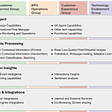# A Global Picture of Algorithmic Problem Solving

“What is computer science?”

Computer science consists of software and hardware; software deals with algorithms and programs that run on hardware. Algorithmic problem solving is fundamental to computer science. “What is algorithmic problem solving?”

What would be your answer to this question, seriously, do not Google it and give the answer from Wiki. Albeit I have been studying computer science and programming for 6 years, it is difficult to answer. A normal and simple version would be: “Oh, algorithmic problem solving is about code algorithms into programs so that we can solve problems that are usually hard to solve by human-level computation power.”

Nothing wrong about this sentence, but can you explain to me, “what are algorithms exactly that you acclaim to be able to solve the problems, what problems exactly are you solving, and what is code?” In my opinion, define and categorize problems, algorithms, and code become the key to depict the global pictures of algorithmic problem solving. Actually, code can be put aside for now, because it is a language, it does not matter what language you use, it only matters what you say. It left us two words to explain: problems and algorithms.

There are numerous books about algorithmic problem solving, but I bet this one is familiar to any one, Introduction to Algorithms, by Thoma Cormen. In this book, Algorithm is defined as any well-defined computational procedure that takes come value, or set of values, as input and produces some value, or set of values, as output. And the book does not really give a concrete definition and classification of problems that algorithm can solve. Working on my own algorithm book, I can not leave these two problems unanswered.

I would define computer science or algorithmic problem solving a multi-disciplined subject, we need to leverage our knowledge in such as math, algebra, geometry, and arithmetic, and so to model the problem and solve the problems related to these subjects.

## Problems

From math, problems are naturally divided into continuous and discrete. On the discrete side, combinatorial problems that are about combining items are more researched. The continuous side seems still remains more in the math, probably, and the representation would be machine learning models that handle the real-number.

## Algorithms

Algorithms are really about problem-solving skills. There are some fundamental principles. From Artificial Intelligence: A Modern Approach, by Stuart Russel, and my observation of course from practice, I draw a conclusion that searching is the fundamental strategy about any problem solving. How could it not be? Algorithms are about to find answer to problem, if and assuming we can define our potential solution space, then a naive searching would do the magic and solve the problem. However, in realty, we are limited by the computation resources, we comprise by:

• be smarter and decrease the cost to find the exact solution we want. This comes down to optimization, which we have divide and conquer, dynamic programming, and greedy algorithms. What are the commonality between them? They all in some way need us to get recurrence relation, which is essentially mathematical induction, which I generalized from another book, Introduction to Algorithms: A Creative Approach, by Udi Manber. Explain it in another way, these principles are using recurrence relation to find the relation of a problem with its smaller instance. Why is it smarter? First, smaller problems are just easier to solve than larger problems. Second, the cost of assembling the answer to smaller problems to answers to the larger problems is possibly smaller.
• by approximating the answer. Instead of trying to get the exact answer, we find one that is good enough. Here goes to all heuristic search, machine learning, artificial intelligence. Guess, currently my limited knowledge is not enough for me to give more context that this.

From my experience, it is so hard to find a book that would be able to give your clear categorization; maybe this field is too complex to have clear classification, so many concepts and intertwined with each other. But, I am just fascinated to find the underlying principle which would be able to guide me better to explore this field. Computer science is not just computer science, it tries to solve all kinds of different problems using computers, it is science and philosophy too, maybe! If I know this, I would stop just looking answer in computer science, look around and search.

--

--

--

## More from Li Yin

Founder@sylphai.com. AI consulting and freelancing. Ex AI researcher@ Meta AI. Github:https://github.com/liyin2015.

Love podcasts or audiobooks? Learn on the go with our new app.

## Google Cloud’s Process Automation Framework for BPOs## Concept Visualization for solving Wumpus World Problem## Can Good Salespeople Ever Be Automated?## Trying different things with GPT-3 Felt Like Witnessing a Technological Revolution## Why Tesla Bot & Amazon Astro show the future of interaction is here## The How of Explainable AI: Pre-modelling Explainability## Li Yin

. AI consulting and freelancing. Ex AI researcher@ Meta AI. Github:.

## Understanding Trees in the world of Computer Science## What does BigO Notation entail? For Beginners: A Simplified Introduction## [Algorithm] Sorting Algorithms -Bubble Sort